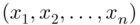# CHAPTER 6PRACTICAL LINEAR ALGEBRA

In the previous chapter, we have introduced the basic and somewhat abstract concepts of linear algebra based on the definition of linear vector spaces and their properties. Here, we introduce the practical side of linear algebra used in many of its real‐life applications. We first discuss linear systems withequations andunknowns in detail. We then introduce row echelon forms, row, column and null spaces, and rank and nullity of matrices. Linear independence, basis vectors, linear transformations and their representations are among the other topics we discuss. Finally, we introduce some of the frequently used numerical methods of linear algebra, where partial pivoting, LU‐factorization, interpolation, and numerical methods of finding solutions are among the topics covered.

## 6.1 SYSTEMS OF LINEAR EQUATIONS

In general, a linear system withequations andunknownsis written as

We can interpret this system as planes in dimensional real space ...

Get Essentials of Mathematical Methods in Science and Engineering, 2nd Edition now with the O’Reilly learning platform.

O’Reilly members experience live online training, plus books, videos, and digital content from nearly 200 publishers.